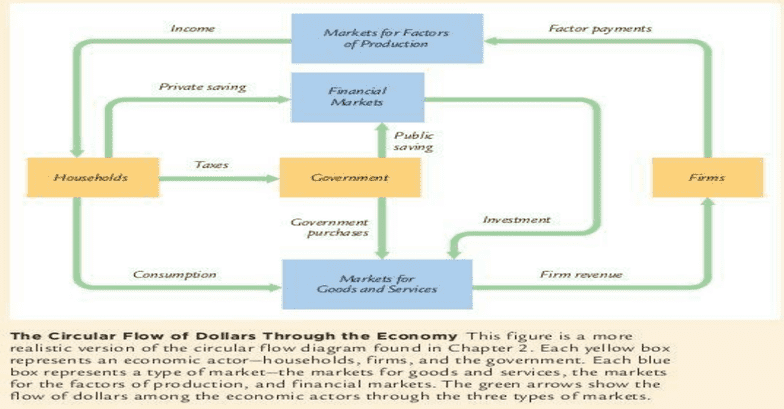Textbook Notes (280,000)
CA (170,000)
UTSC (20,000)
Chapter 3

# MGEB06H3 Chapter Notes - Chapter 3: Profit Maximization, Marginal Product, Real Wages

Department
Economics for Management Studies
Course Code
MGEB06H3
Professor
Iris Au
Chapter
3

This preview shows page 1. to view the full 4 pages of the document.CHAPTER 3 NATIONAL INCOME: WHERE IT COMES FROM AND WHERE IT GOES
- How much do firms in the economy produce? What determines a nation’s total income?
- Who gets the income from production? How much goes to compensate workers and how much goes to compensate owners of
capital?
- Who buys the output of the economy? How much do households purchase for consumption, how much do households and
firms purchase for investment, and how much does the government buy for public purposes?
- What equilibrates the demand for and supply for goods and services? What ensures that desired spending on consumption,
investment, and government purchases equals the level of production?
Circular flow diagram:
WHAT DETERMINES THE TOTAL PRODUCTION OF GOODS AND SERVICES?
1. The quantity of inputs (factors of production)
2. Its ability to turn input into output
FACTORS OF PRODUCTION
Most important: capital (K) and labour (L)
__ __
K = fixed amount of capital L = fixed amount of labour
PRODUCTION FUNCTION: Y = F(K, L)
Y= amount of output
Function reflects available technology for turning capital and labour (thus tech change alters the production function)
Many production functions have a property called constant returns to scale:
- Has constant returns to scale if an increase of an equal percentage in all factors cause an increase in output of the same
percentage
o Mathematically: zY = F(zK, zL) z = any positive number
Equation state: if we multiply both the amount of capital and the amount of labour by some number z, output is also
multiplied by z
_ _ _
SUPPLY OF GOODS AND SERVICES Y = F(K, L) = Y
Meaning: determine the quantity of goods and services supplies, in turn equals to the economy’s output
HOW IS NATIONAL INCOME DISTRIBUTED TO THE FACTORS OF PRODUCTION?
Modern theory of how national income is divided among the factors of production
- based on the classical (18th century) idea that prices adjust to balance supply and demand